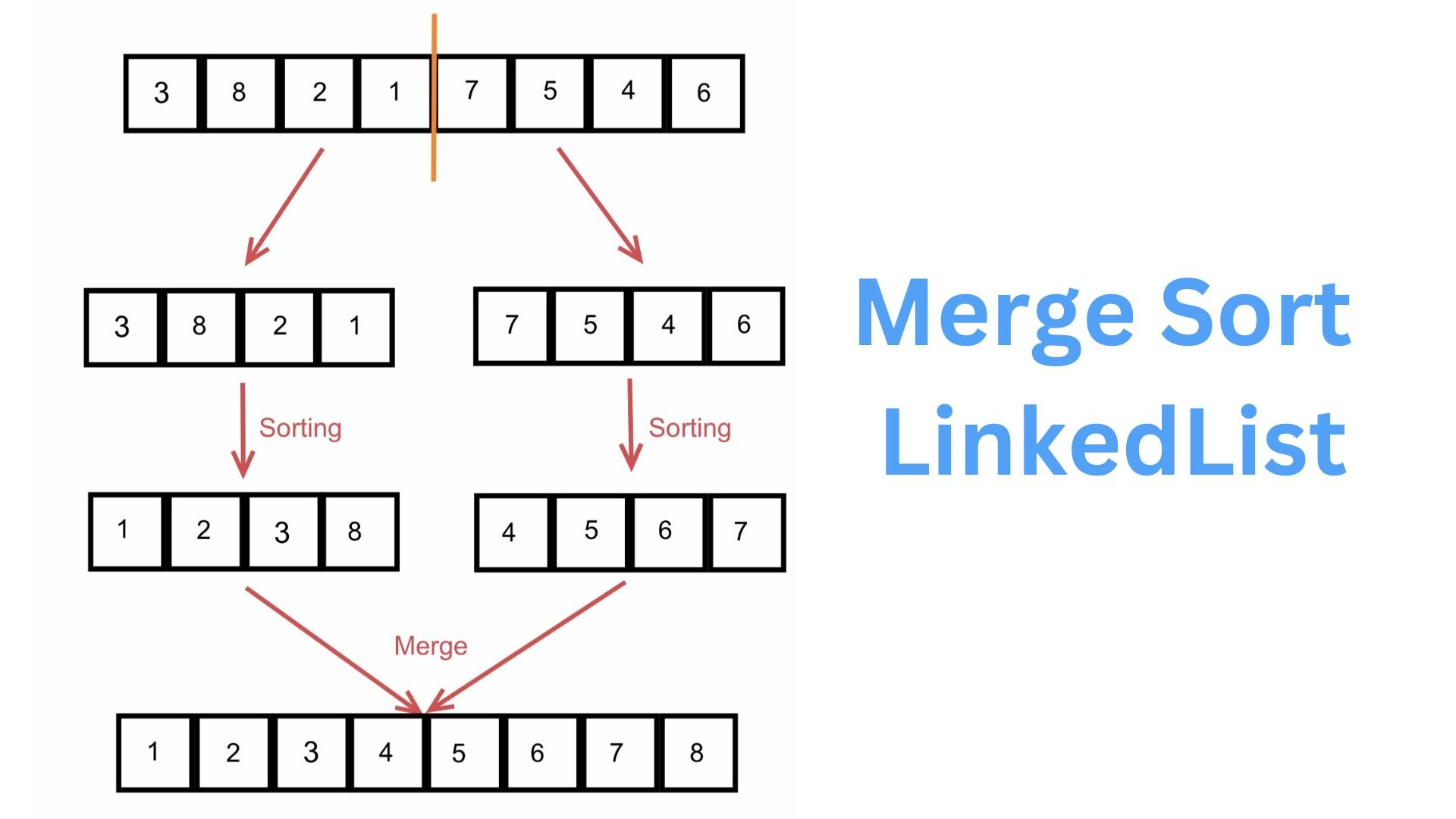# How to use Merge Sort with Linked Lists## Introduction

Sorting is the process of putting items in order that may be either ascended or ascended. There are several sorting algorithms with varying temporal complexity that may be used to quickly arrange data. This post will teach us how to merge sort for linked lists. Problem statement for the linked list's merge sort The issue description states that we are given a singly linked list and that we must sort it using merge sort. An algorithm that uses divide and conquer is merge sort.

## Features of Merge Sort

• This algorithm is recursive.
• To merge sort, we must split the container (which might be an array, list, etc.) into two parts before using merge sort recursively on the two parts.
• These two merge sort calls yield a sorted container, which we then merge to keep the container's overall sorting.
• See how to merge sort functions in a nutshell by looking at the graphic below.

Now that we've learned a little bit about the merge sort algorithm. Let's look into merging sorting for linked lists. In order to apply merge sort to a linked list, we must first recursively split the list into two sub-lists at each step until the list size is reduced to one. Once we have returned from the recursive call, we have two sorted lists that will be merged into a single list by the merge operation in linear time. We will now examine the method and technique used to perform merge sort on linked lists.

## Method and Algorithm for Linked List Merge Sorting

1. The size of our linked list is either 1 or 0, and a linked list of size 0 or 1 is already sorted if the head of the linked list is NULL or (head next == NULL). Therefore, do nothing and simply turn around.
2. Find the middle of the linked list first if the linked list's size is greater than 1.
• Use both the slow and fast pointer methods to locate the center node.
• We take two pointers, slow and fast, and initialize them with head in this procedure.
• Once the fast pointer reaches the end of the list, we shift the slow pointer by one node and the fast pointer by two nodes.
• The slow pointer will be in the middle of the linked list when the fast pointer reaches the tail.
• When the fast pointer reaches the tail, the slow pointer will only have traversed half of the list because it is moving at half the speed of the fast pointer.
• This is the only reason why the slow pointer will be in the middle of the list.
1. Next, assign slow next = NULL to the pointer afterMiddle where you previously stored slow next.
2. Recursively run mergeSort() on the left and right sub-linked lists, and then store the new heads of the left and right linked lists in the pointer variables part1 and part2, respectively.
3. Merge the two linked lists that are returned by the recursive calls on the left and right sub-lists.

## Algorithm for combining two sorted linked lists:

We will combine the two sorted lists that the two recursive calls will return into a single list using the procedures listed below.

1. Set the left and right sorted sublists as the initial values for the two pointer variables curr1 and curr2, respectively.
2. The head and tail of the final sorted linked list are represented by the two pointer variables si and ei, which should be initialized with NULL. Store Curr1 in the next of ei and transfer
3. Curr1 to the next of Curr1 if Curr1's data is smaller than Curr2's data. Otherwise, put Curr2 in the next of EI and transfer Curr2 to the next of Curr2 if the Data of Curr2 is smaller than the Data of Curr1.
4. Continue performing steps 3 and 4 until neither curr1 nor curr2 is equal to NULL. The remaining nodes from the first or second linked lists should now be added to the combined linked list.
5. The head of the combined sorted linked list, which includes every node from the two sorted sub-lists, should be returned.